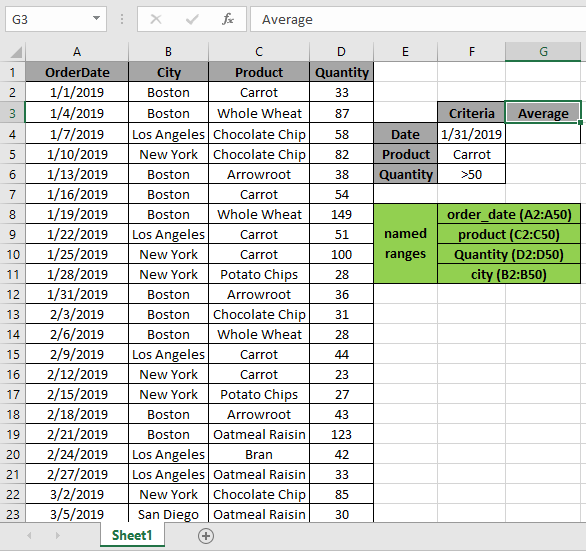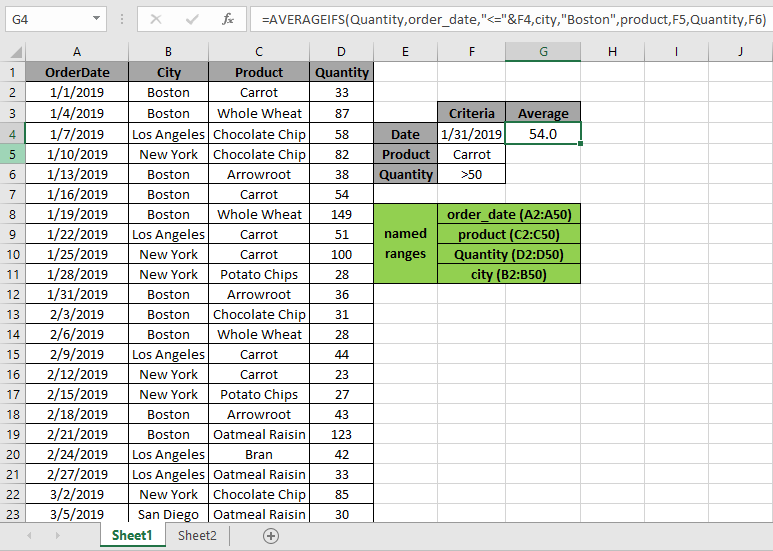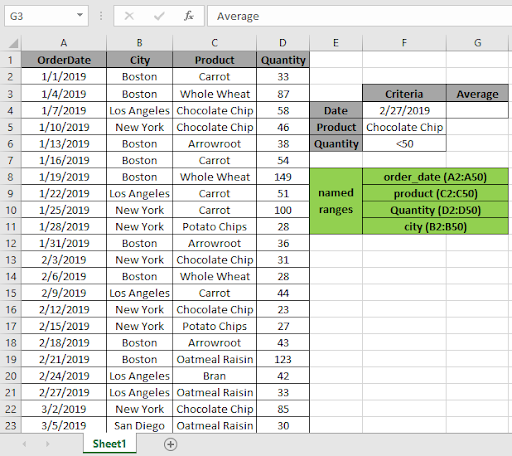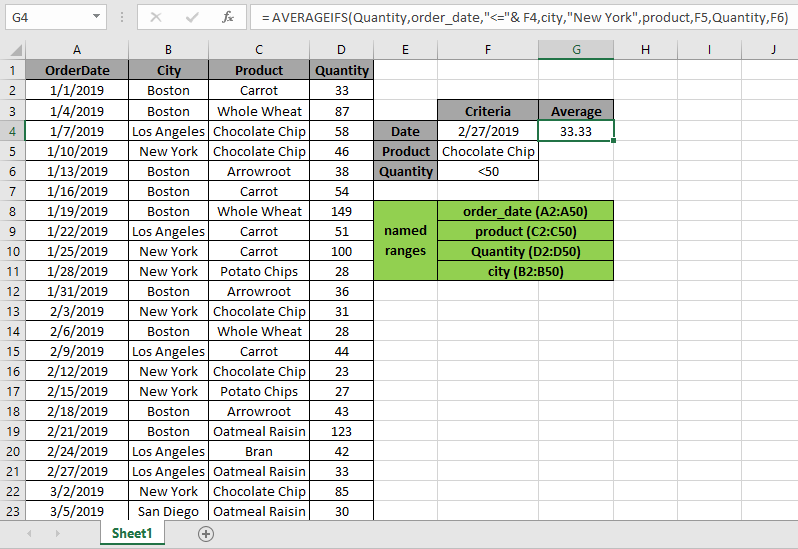# How to Find Average Based on Multiple Criteria in Microsoft Excel 2010

In this article, we will learn how to get the Average with multiple criteria in Excel.

Problem?

For Instance, We have a large list of data and we need to find the Average of the price or amount given some criteria. Criteria can be applied over any column of the data.

How to solve the Problem.

Now we will make a formula out of the function. Here we are given the data and we needed to find the AVERAGE of the numbers having some criteria

Generic formula:

 = AVERAGEIFS ( Average_range , range1, criteria1, [ range2, criteria2 ], [ range3, criteria3 ], … )
• Average_range : range of values where average needs to be evaluated
• range1 : first range where criteria1 is applied.
• criteria1 : first criteria applied on range1.
• range2 : second range where criteria1 is applied.
• criteria2 : second criteria applied on range2.
• range3 : third range where criteria1 is applied.
• criteria3 : third criteria applied on range3.

Notes: do not provide date directly to the function. Use DATE function or use cell reference for date argument in excel as Excel reads date only with the correct order.

Example:

All of these might be confusing to understand. So, let's test this formula via running it on the example shown below.

Here we have given data from A1:D51. We need to find the AVERAGE of Quantity received as per different criteria.

Conditions are as follows.

1. Date must be before 1/31/2019.
2. City "Boston"
3. Product be defined as "Carrot"
4. Quantity must be greater than 50 ( >50 ).The above 4 stated conditions or criteria needs to be matched. The AVERAGEIFS function can help us extract the average having different criteria.

Use the Formula:

 = AVERAGEIFS ( Quantity , order_date , "<=" & F4 , city , "Boston" , product , F5 , Quantity , F6 )

Quantity : named range used for D2:D50 array

Order_date : named range used for A2:A50 array

City : named range used for B2:B50 array

Product: named range used for C2:C50 array

F4 : cell reference used for criteria 1

F5 : cell reference used for criteria 2

F6 : cell reference used for criteria 3

Explanation:

1. order_date , "<=" & F4  first criteria which suggests date before 1/31/2019 in F4.
2. city , "Boston" second criteria where city matches "Boston"
3. product , F5 where product matches Carrot in F5 cell
4. Quantity, must be under criteria in F6 cell i.e greater than 50.

Here the A2 is given as cell reference & Named ranges given as rng ( D2 : D51 ) and order_date ( A2 : A51).As you can see in the above snapshot the AVERAGE of the quantity in January comes out to be 54.00. As there is only one value satisfying all conditions.

Example 2:

Here we have given data from A1:D51. We need to find the AVERAGE of Quantity received as per different criteria.

Conditions are as follows.

1. Date must be before 2/27/2019.
2. City "New York"
3. Product be defined as "Chocolate Chip".
4. Quantity must be less than than 50 ( < 50 ).The above 4 stated conditions or criteria needs to be matched. The AVERAGEIFS function can help us extract the average having different criteria.

Use the Formula:

 = AVERAGEIFS ( Quantity , order_date , "<=" & F4 , city , "New York" , product , F5 , Quantity , F6 )

Quantity : named range used for D2:D50 array

Order_date : named range used for A2:A50 array

City : named range used for B2:B50 array

Product: named range used for C2:C50 array

F4 : cell reference used for criteria 1

F5 : cell reference used for criteria 2

F6 : cell reference used for criteria 3

Explanation:

1. order_date , "<=" & F4  first criteria which suggests date before 2/27/2019 in F4 cell.
2. city , "Boston" second criteria where city matches "Boston"
3. product , F5 where product matches Carrot in F5 cell
4. Quantity, must be under criteria in F6 cell i.e less than 50.

Here the A2 is given as cell reference & Named ranges given as rng ( D2 : D51 ) and order_date ( A2 : A51).As you can see in the above snapshot the AVERAGE of the quantity in January comes out to be 33.33... As there is only one value satisfying all conditions.

Here are some observations about the formula usage below.

Notes:

1.  The function returns #VALUE! error if the argument to the function is non-numeric.
2. The function returns #VALUE! error if no value matches the given criteria.
3. The formula accepts operators such as =, < , <= , >= , <= & <>.
4. Do not provide date argument directly to the function. Use DATE function or use cell reference for date argument in excel as Excel reads date only with the correct order.
5. The function returns #VALUE! criteria if no value matches the given criteria.
6. Different Criteria inside formula is accepted, such as equals to (=), less than (<) , less than or equals to (<=) , greater than or equals to (>=) , greater than (>) & not equals to (<>).

In this way, we learnt How to Find Average Based on Multiple Criteria in Microsoft Excel 2010. You can use these functions in Excel 2016, 2013 and 2010. Find more articles on Mathematical formulation with different criteria. If you liked our blogs, share it with your friends on Facebook. And also you can follow us on Twitter and Facebook. We would love to hear from you, do let us know how we can improve, complement or innovate our work and make it better for you. Write us at info@exceltip.com

Related Articles

How To Highlight Cells Above and Below Average Value : highlight values which are above or below the average value using the conditional formatting in Excel.

Ignore zero in the Average of numbers : calculate the average of numbers in the array ignoring zeros using AVERAGEIF function in Excel.

Calculate Weighted Average : find the average of values having different weight using SUMPRODUCT function in Excel.

Average Difference between lists : calculate the difference in average of two different lists. Learn more about how to calculate average using basic mathematical average formula.

Average numbers if not blank in Excel : extract average of values if cell is not blank in excel.

AVERAGE of top 3 scores in a list in excel : Find the average of numbers with criteria as highest 3 numbers from the list in Excel

Popular Articles:

How to use the IF Function in Excel : The IF statement in Excel checks the condition and returns a specific value if the condition is TRUE or returns another specific value if FALSE.

How to use the VLOOKUP Function in Excel : This is one of the most used and popular functions of excel that is used to lookup value from different ranges and sheets.

How to use the COUNTIF Function in Excel : Count values with conditions using this amazing function. You don't need to filter your data to count specific values. Countif function is essential to prepare your dashboard.

How to use the SUMIF Function in Excel : This is another dashboard essential function. This helps you sum up values on specific conditions.

Terms and Conditions of use

The applications/code on this site are distributed as is and without warranties or liability. In no event shall the owner of the copyrights, or the authors of the applications/code be liable for any loss of profit, any problems or any damage resulting from the use or evaluation of the applications/code.